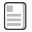# That Blue Square Thing

## AQA Computer Science GCSE

This page is up to date for the AQA 8525 syllabus for exams from 2022.

### Programming Concepts - Selection

Selection is one of the core programming ideas. It uses IF statements to allow a program to go down different paths depending on the value held in a variable of some kind.

IF statements are absolutely crucial to the flow of a program. Without them programs would always follow the same route.

#### Simple IF blocks:

IF blocks are simple enough to use. You just need a value of some kind to test.

IF myName = "Clive" THEN

# do whatever code is here

ENDIF

Note that in Pseudocode we need to add an ENDIF statement at the end of the block, whereas in Python we don't:

if myName == "clive":

# do whatever code is here

# and then outdent the code again

Note that in the two examples I used "Clive" and then "clive" as the test. These aren't the same thing - there is a difference between a string using a capital letter and one that doesn't.

#### IF–ELSE blocks:

Sometimes you want to do something different if the IF test is not true. That's easy to do using an IF–ELSE block.

IF myAge < 18 THEN
OUTPUT "You are too young to vote"
ELSE
OUTPUT "You are old enough to vote"
ENDIF

Again, in Python we don't need an ENDIF statement::

if myAge < 18:
print("You are too young to vote")
else:
print("You are old enough to vote")

IF–ELSE works fine if there are only two possible options.

#### ELSE–IF blocks

Sometimes there are more than one possible outcome of a set of tests. ELSE–IF can be a good way to deal with this issue.

This works well if there are a set of possible outcomes all based on the same variable. For example, a set of grades for an exam:

IF theScore > 80 THEN
OUTPUT "You got an A"
ELSE IF theScore > 70 THEN
OUTPUT "You got a B"
ELSE IF theScore > 60 THEN
OUTPUT "You got a C"
ELSE
OUTPUT ("You got a U")
ENDIF

In Python ELSE–IF becomes elif. That simply means "Else, if this is True".

if myScore > 80:
print("Well done, you got an A grade")
elif myScore > 70:
print("That's good, you got a B grade")
elif myScore > 60:
print("That's fine, you got a C grade")
else:
print("Oh dear, you failed")

The way that elif works is really clever and it's worth taking 2 minutes to try to understand it.

It works down the block until if finds the first thing that's True. Then it does that. So, if you got a score of 75, the program would first test whether you got >80. No, you didn't, so it skips to the next possible test - did you score > 70. yes you did, so it executes whatever commands are linked to this and then skips the rest of the block entirely.

Crucially, this means that it doesn't print that you got a C. Although 75 is > 60, it's already found a section that's True and so it can skip the rest of the elif statements.

This makes elif much more powerful than using three separate IF statements - and much easier to code.

Note that the else statement at the end is whatever you want to happen if none of the other things are True. You don't need to include a final else statement - although this might mean that nothing happens (which is sometimes what you want).

#### Nested IF statements:

Nested IF statements are when you place an IF block inside of another IF block. They can be used in all sorts of ways - to test two different variables together or to do similar things to ELIF blocks.

So, for example...

IF gender = "F" THEN
IF age >= 18 THEN
OUTPUT "Hello Mrs " + theSurname
ELSE
OUTPUT "Hello Miss " + theSurname
ENDIF
ELSE
IF age >= 18 THEN
OUTPUT "Hello Mr " + theSurname
ELSE
OUTPUT "Hello Master " + theSurname
ENDIF
ENDIF

The Python code for this is straightforward enough - just be careful where the indents go.

You can also use Nested IF blocks in place of ELIF:

IF theScore > 80 THEN
OUTPUT "You got an A"
ELSE
IF theScore > 70 THEN
OUTPUT "You got a B"
ELSE
IF theScore > 60 THEN
OUTPUT "You got a C"
ELSE
OUTPUT ("You got a U")
ENDIF
ENDIF
ENDIF

This is much more complex than using the ELSE–IF method, but sometimes you need to go down a Nested IF route to get the outcome you require. The Python code is below so you can test this against the same logic as the ELIF - and I'll throw in a program you can download as well, so that you can compare the two methods at work.

if theScore > 80:
print("You got an A.")
else:
if theScore > 70:
print("You got a B.")
else:
if theScore > 60:
print("You got a C.")
else:
print("You got a U.")

You really have to watch your indent levels with nested IF blocks. This is tricky - if ELIF is an option you're better off using it.Different IF approaches - program code - open the text file, copy the contents into a new IDLE (Python) window and save before running